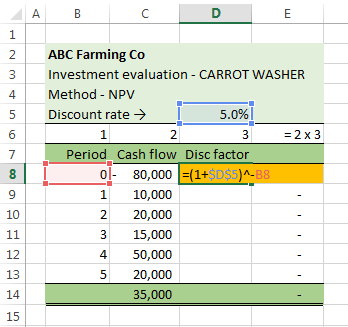# Reference to cells

## Reference styles

A reference style is determined by the address of the home cell (the top left cell in a worksheet). Excel has two styles, the A1 reference style and the R1C1 reference style. You can download the workbook for this module from the link on the figure 6 Excel Web App #1.

### A1 reference style

• The header row has alphabetic characters {A, B, C, …, XFB, XFC, XFD}
• The address of the home cell is the intersection of column A and row 1, denoted A1 – see figure 1 – left panel
• To goto the Home cell, use the Ctrl + Home shortcut
• A1 reference style is (column, row) notation
• This is the default style and the most commonly used spreadsheet reference style
• The style was inherited from Lotus 1-2-3, and VisiCalc
• Trivia: It is also the reference system for street directories such as the Australian Melway directory

### R1C1 reference style

• The header row has numerical characters {1, 2, 3, …, 16382, 16383, 16384}
• The address of the home cell is the intersection of row 1 and column 1, denoted R1C1 – see figure 1 – right panel
• To goto the Home cell, use the Ctrl + Home shortcut
• R1C1 reference style is (row, column) notation
• The style is also used in mathematics, and numerical computing where elements of a matrix or array are indexed with (r,c) notation
• A number of Excel functions use row, and column references – such as the INDEX and OFFSET functions

### Switching styles

To change styles, use the ribbon sequence Home > Options > Formulas > Working with formulas, then check / uncheck the R1C1 reference style box.

## Cell references

### Relative reference

The type of cell reference is important when:

• Using references in a formula, and
• The formula is copied to other cells
• An example of relative referencing is shown in figure 2
• Copies of all example worksheets are available in the Excel Web App #1 – see figure 6
1. The formula in cell Relative!D7 $$\left( 1+r \right)^{-t}$$ has an exponent value from cell B7. D7: =(1+5%)^-B7
2. Relative to D7, the cell B7 is in the same row and 2 columns to the left
3. In R1C1 style, the formula in cell D7 is =(1+5%)^-RC[-2]. In the same row R and 2 columns left C[-2]
4. When the D7 formula is copied down the column, the cell D8 formula is =(1+5%)^-B8
5. In R1C1 style, the formula in cell D8 is =(1+5%)^-RC[-2]. The same as D7
6. An example of R1C1 relative addressing is shown in figure 3
• ### Absolute reference

The ABC Farming Co carrot washer example is continued. This time the discount rate is assigned to a cell (Absolute!D5), rather than being entered as a formula constant.

•Fig 4: Absolute reference A1 style – used for the discount rate reference of the formula
1. The formula in cell Absolute!D8 has an exponent value from cell B8 (relative), but the discount rate is an absolute reference to cell D5 as shown by the $signs. =(1+$D$5)^-B8 2. When the D8 formula is copied, the discount reference will always refer to the cell D5 3. In R1C1 style, the formula in cell D8 is =(1+R5C4)^-RC[-2] 4. When the D8 formula is copied down the column, the cell D9 formula is =(1+$D$5)^-B9 5. In R1C1 style, the formula in cell D9 is =(1+R5C4)^-RC[-2]. The same as D8 ### Mixed reference The discounted cash flow theme is continued. This example constructs a table of discount rate for present value (PV). A framework is shown in figure 5, and Excel Web App #1 worksheet Mixed. The idea is to enter one formula in cell C4, and then copy this formula to the range C4:L24. •Fig 5: Mixed reference A1 style – used in the construction of a two dimensional array of discount factors 1. The formula in cell Mixed!C4 has an exponent value reference to cell$B4 (absolute column and relative row, a mixed reference), and the discount rate reference to cell C$3 (relative column and absolute row, a mixed reference). C4 =1/(1+C$3)^\$B4
2. When the C4 formula is copied D4, the discount reference will always refer to the cell in row 3 and the same column as the formula. The period reference will always refer to the cell in column B and the same row as the formula
3. When the C4 formula is copied C5, the discount reference will always refer to the cell in row 3 and the same column as the formula. The period reference will always refer to the cell in column B and the same row as the formula
4. In R1C1 style, the formula in cell C4 is =1/(1+R3C)^RC2. When copied this same formula will be in every cell of the body of the Table of Discount Factors

### Reference shortcuts

• In edit mode, pressing the F4 key cycles through the four combinations of relative and absolute referencing
• Press F4 before adding the closing bracket, next operator, or comma separator. Otherwise select the reference in equation edit mode, and cycle with F4 to the desired cell reference combination
• This example was developed in Excel 2013 Pro 64 bit.
• Last modified: 24 Nov 2017, 6:12 am [Australian Eastern Time (AET)]
• Thanks to Tom for comments about the Mixed reference section [12 Nov 2017]